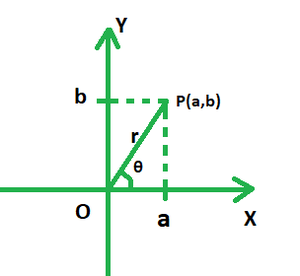Open In App

# Find the fourth roots of 5(1 + i√3)

Real and imaginary numbers combine to form complex numbers. The imaginary component, I (iota), indicates a square root of -1. The imaginary portion of a complex number is i. a + ib is a typical representation of complex numbers in their rectangular or standard form. For example, 100 + 25i is a complex number in which 100 represents the real part and 25i represents the imaginary part.

### Polar Form Representation of Complex Numbers

To represent a complex number, the polar coordinates of the real and imaginary components are written here. represents the angle at which the number line is inclined to the real axis, i.e. the x-axis. The length indicated by the line is known as its modulus, and it is represented by the letter r in the alphabet. The real and imaginary components are represented by a and b, respectively, while the modulus is represented by OP = r in the diagram below.The Pythagoras theorem is to be used to get the length r. Trigonometric ratios can be used to calculate arguments. The polar form of a complex number of the type z = a + ib is represented as follows:

r = Modulus[cos(argument) + isin(argument)]

Alternatively, z = r[cosθ + isinθ]

In this case, r =and θ = tan-1{b/a}.

Calculating Roots of Complex Numbers

DeMoivre’s Theorem can be used to simplify higher-order complex numbers. It can be used to determine the roots of complex numbers as well as expand complex numbers according to their exponent.

Given:, then its roots are:Where, k lies between 0 and n – 1 and n is the exponent or radical.

### Find the fourth roots of 5(1 + i√3). Leave in trigonometric form.

Solution:

Modulus of the given number == 10

Argument = tan-1[5√3/ 5] = π/3.

Thus, the polar form of 5 + 5√3i =According to DeMoivre’s formula, all the nth roots of a complex number are given by:, where  k lies between 0 and n – 1 and n is the exponent or radical.

Here, r = 10, θ = π/3 and n = 4.

Find the 4 roots by substituting the values of k as 0, 1, 2 and 3 respectively.

• For k = 0, z =• For k = 1, z =• For k = 2, z =• For k = 3, z =Thus, the four roots of 5(1 + i√3) areand.

### Similar Problems

Question 1: Find the cube roots of -2 – 2√3i.

Solution:

r == √(16) = 4, θ = 4π/ 3.

According to DeMoivre’s formula, all the nth roots of a complex number are given by:, where k lies between 0 and n – 1 and n is the exponent or radical.

Find the 3 roots by substituting the values of m as 0, 1 and 2 respectively,

For m = 0, z == 0.27 + 1.56i

For m = 1, z == −1.49 − 0.54i

For m = 2, z == 1.21 −1.02i

Thus, the roots are 0.27 + 1.56i, −1.49 − 0.54i and 1.21 – 1.02i.

Question 2: Find the fifth roots of 32 + 0i.

Solution:

Modulus == 32.

Argument = θ = tan-1(0/ 32) = 0.

According to DeMoivre’s formula, all the nth roots of a complex number are given by:, where k lies between 0 and n – 1 and n is the exponent or radical.

Find the 5 roots by substituting the values of m as 0, 1, 2, 3 and 4.

For m = 0,= 2

For m = 1,

z == 0.62 + 1.9i

For m = 2,

z == −1.62 + 1.18i

For m = 3,= −1.62 − 1.18i

For m = 4,= 0.62 − 1.9i

Thus, the roots are 2, 0.62 + 1.9i, -1.62 + 1.18i, -1.62 – 1.18i and 0.62 – 1.9i.

Question 3: Find the fourth roots of -8√3 + 8i.

Solution:

Polar form =We have k = 2, n = 4 and θ = 5π/ 6.

According to DeMoivre’s formula, all the nth roots of a complex number are given by:, where k lies between 0 and n – 1 and n is the exponent or radical.

Find the 4 roots by substituting the values of m as 0, 1, 2 and 3 respectively.

For m = 0,For m = 1,For m = 2,For m = 3,Thus, the four roots of z are 1.58 + 1.21i, −1.21 + 1.58i, −1.58 −1.21i and 1.21 − 1.58i.

Question 4: Find the sixth root of -27i. Leave in trigonometric form.

Solution:

Modulus =Argument = θ = tan-1(-27/ 0) = π/2.

Polar form =According to DeMoivre’s formula, all the nth roots of a complex number are given by:, where k lies between 0 and n – 1 and n is the exponent or radical.

Find the 6 roots by substituting the values of m as 0, 1, 2, 3, 4 and 5.

• For m = 0,  z =• For m = 1,  z =• For m = 2,  z =• For m = 3,  z =• For m = 4,  z =• For m = 5, z =Question 5: Find the fourth root of 81i. Leave in trigonometric form.

Solution:

Polar form =We have k = 81, n = 4 and θ = π/ 2.

According to DeMoivre’s formula, all the nth roots of a complex number are given by:, where k lies between 0 and n – 1 and n is the exponent or radical.

Find the 4 roots by substituting the values of m as 0, 1, 2 and 3 respectively.

• For m = 0, z ==• For m = 1, z ==• For m = 2, z ==• For m = 3, z =